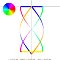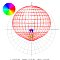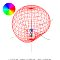Home
Antennas
QFH
Simulation

Language:
Español
Deutsch

Simulations
Dimensions
Groundplane

Related:
Simple helicoidal

Simulation of several versions

 Description W/H=0.32 W/H=0.38 W/H=0.44 W/H=0.50 W/H=0.44inv Geometry (X-axis)Geometry (3D)Radiation diagram (X-axis)Radiation diagram (Y-axis)Radiation diagram (Z-axis)Radiation diagram (3D)Radiation diagram (lhcp)Radiation diagram (rhcp)Input file for NEC20.32 W/H Ratio = 0.32 0.38 W/H Ratio = 0.38 0.44 W/H Ratio = 0.44 0.50 W/H Ratio = 0.50 0.44inv W/H Ratio = 0.44, loops wrongly connected (inverted)

 (c) John Coppens ON6JC/LW3HAZ mail